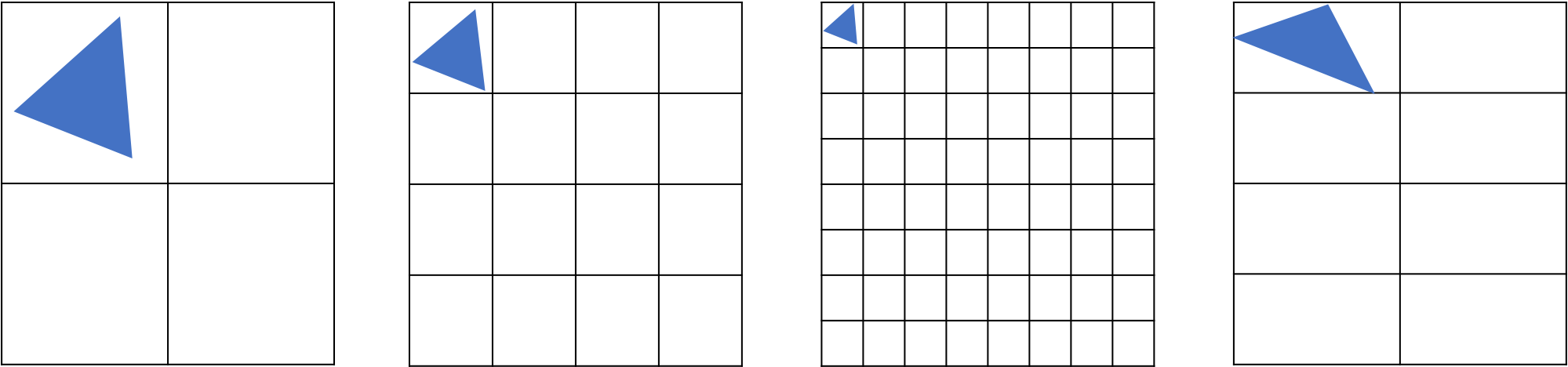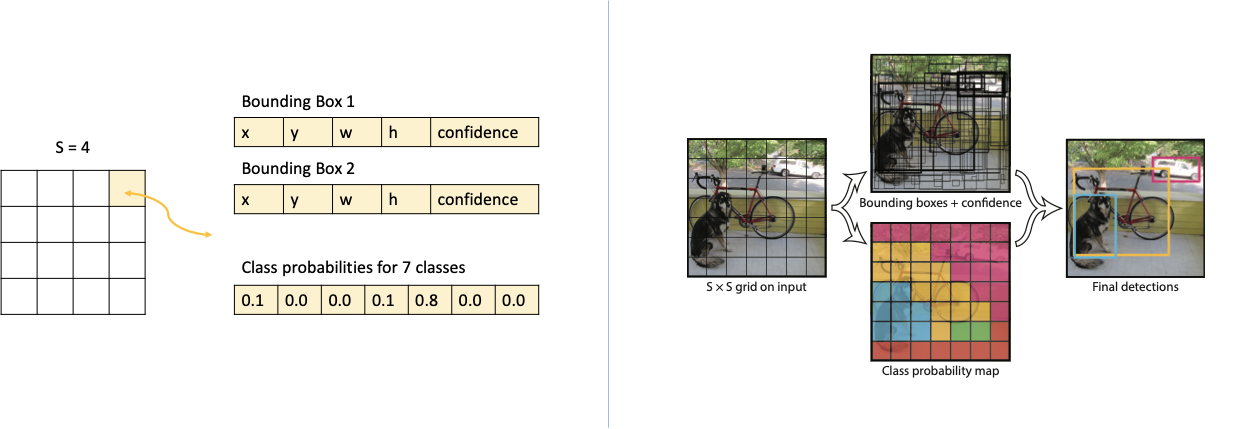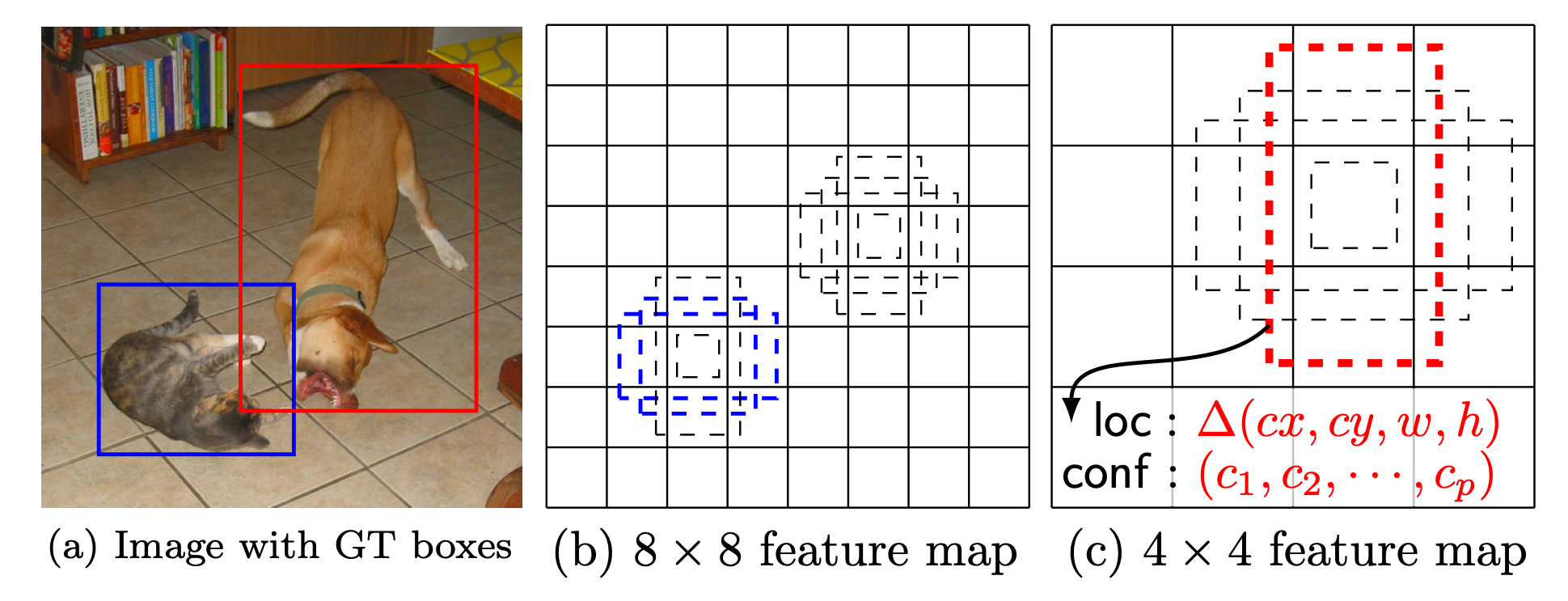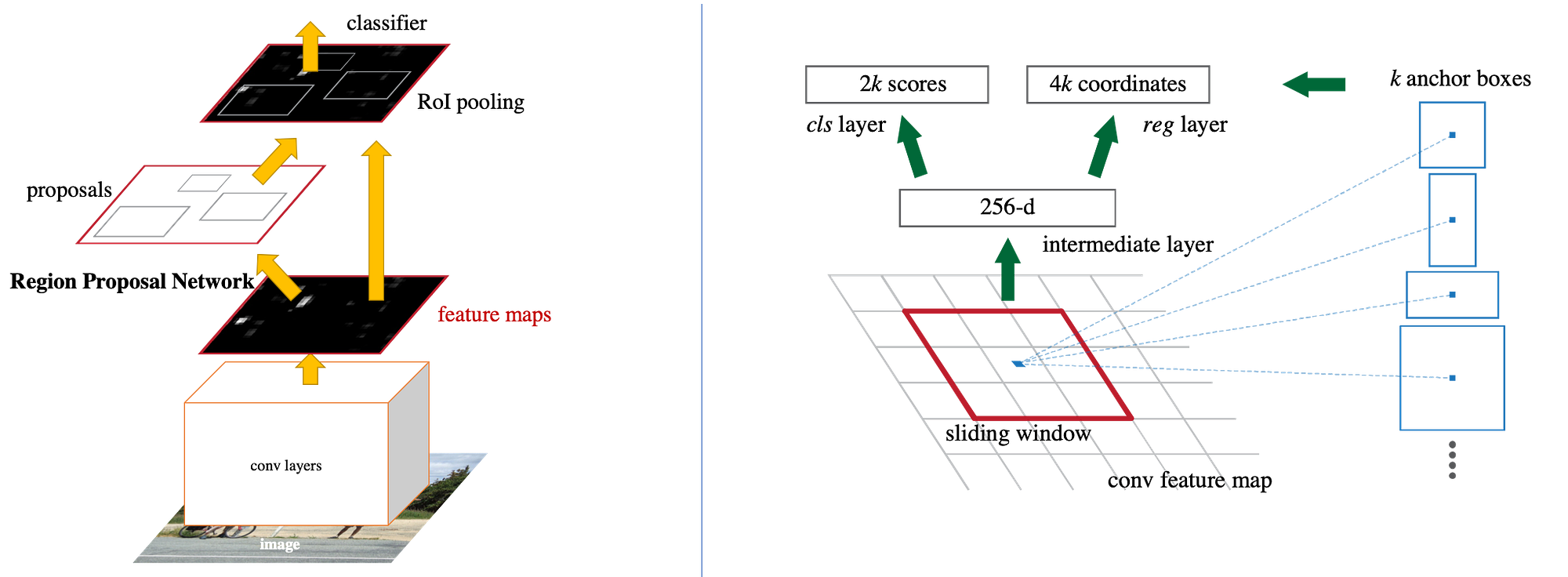# Object Detection – A Look At Yolo, SSD, Faster RCNN

While image classification capabilities have many uses, most real world applications require more than classification of singleton images. We need the ability to detect multiple objects in a given image.

# Getting Started

Basic image classification models are relatively straight forward. Given an image as input, the goal is to classify it as one of the many possible output classes. The architecture is typically, but not necessarily, a series of Convolution and Pooling layers flattened at the end with one or more linear layers with the final output layer having the same number of nodes/neurons as the number of classes. Each node in the output layer represents a class and the model will be trained for this final layer to give the probabilities that a given input image belongs to the corresponding class.

The illustration above is of a multi class image classification example where the outputs are mutually exclusive, i.e. all the output probabilities sum-up to 1. Another variation is multi label classification where each probability is independent of the other and an image could be tagged to more than one class, like associating genres to movie posters.

# Goals

While such image classification capabilities have many applications, most real world applications require more than such classification of singleton images. If you think of self driving cars as an example (NOTE: the real self driving solutions are likely more sophisticated with nuances, but go with this example for illustrative purposes), it requires us to:

1. Determine the position of the identified object in the image. For example: if the identified pedestrian is right in front or to the side
2. Identify more than one object. For example: a single image could have multiple cars, many pedestrians, traffic light, etc
3. Identify the orientation of the object. For example: the front of the car is facing towards and rear facing away (i.e. car is coming towards us or parked facing us)

Accomplishing all this requires a little more to be done than the image classification models. In this post, we will look at accomplishing the first two goals.

# A thought exercise

So how do we determine the position, defined by bounding box coordinates, of the object(s) in addition to classification? And how do we determine multiple objects when present?

The way we want to determine the position is by identifying the coordinates of the bounding box of the objects. Our goal will be to get the coordinates of the bounding boxes, typically by identifying the coordinates of the corner or center along with the height and width of the bounding box.

Side Note: Another alternative is to get the masks of the identified object instances. For the identified object, the mask gives us all the pixels that are part of identified instance. Fig 1 illustrates both of these output options (a multi object detection illustration is used for ease understanding). The dotted lines represent the bounding box output whereas the colored objects represents the mask output we want to get given an input image.

Let’s do a simple thought exercise starting with a couple of constraints that will simplify the thinking and come back to remove them later. One, let’s assume all objects are of a fixed width and height (say 20px * 20px). Two, let’s assume these objects will start at 0 or multiples of 20. i.e. their top and left coordinates will be either 0 or multiples of 20 (i.e. top left will be 0,0 or 0,20 or 20,40 or 40,40 etc).

With these two constraints in place, one way to determine the exact position of the object would be to imagine a grid across the image such that each cell is of size 20*20. Now, all we have to do is for each cell in the grid evaluate the class probabilities like in image classification. And all cells that have a class probability higher than a threshold, are where the objects are!

Now when we remove the two constraints of predetermined size and position, it should become evident that somehow we have to have a grid system that helps us determine boxes of various sizes, aspect ratios and positions. These boxes are sometimes called the anchor boxes. There are different approaches on how to address this problem of generating varying sized/positioned anchor boxes, and we will look at how some of the well known approaches.

It is also important to note that in this simple thought exercise, we imagined the grid system and anchor boxes directly over an image, but technically we will do this on a feature map representing the image like the last layer of a base network through which the image is passed.Fig 2: Detecting the bounding box requires evaluating boxes of different sizes, aspect ratios and positions

## YOLO

Yolo (paper link) for example divides the input image into an S × S grid. Each grid cell is evaluated for not only the class probabilities as we did above, but a set of “B” bounding boxes and confidence scores for those boxes are also predicted alongside.

In other words, the boxes are not predetermined like in our simple thought exercise, but are predicted along with the class probabilities with the cell. Each bounding box consists of 5 predictions: x, y, w, h, and confidence. The first four pertains to coordinates, the last one, confidence reflects how confident the model is that the box contains an object and how accurate the box coordinates are.

In addition, the responsibility of determining the box coordinates of an object belongs to the grid cell in which the center of the object falls into. This helps prevent multiple cells determining boxes around the same object. But each cell still predicts multiple bounding boxes. One of these boxes is deemed “responsible” for predicting an object based on which prediction has the highest current IOU with the ground truth during training. This during the course of training results in the different bounding boxes in each cell specializing at predicting certain sizes, aspect ratios, or classes of object, improving overall recall.

These predictions are encoded as an S × S × (B ∗ 5 + C) tensor (S x S is the grid dimension, B is the boxes each cell in the grid will determine, 5 is the predictions made per box namely x, y, w, h and confidence, C is the probabilities for the C classes of objects the model can identify).Fig 3: Yolo illustrations, second part from the original paper here. On the left is an illustration of a 4 x 4 grid generating two bounding boxes and 7 class probabilities.

## SSD

Single Shot MultiBox Detector (SSD) (paper link), doesn’t predict the boxes out of nothing, but starts with a set of default boxes. It uses several feature maps of different scales (i.e. several grids of different sizes like 4 x 4, 8 x 8 etc as seen in Fig 4) and a fixed set of default boxes of different aspect ratios per cell in each of those grids/feature maps. For each default box, the model then computes the “offsets” along with the class probabilities. The offsets are set of 4 numbers cx, cy, w and h — giving the offset of the center coordinates and width and height of the real box with respect to the default box.

SSD also differs in its strategy to match the object ground truth boxes to the default boxes. There is no single default box held responsible for and matched to an object. Instead default boxes are matched to any ground truth with IOU higher than a threshold (0.5). This means high scores will be predicted for multiple default boxes overlapping with the object, rather than requiring holding just one of those boxes responsible.Fig 4: SSD Framework from the original paper here. Illustrates multiple (two) blue boxes matching the cat and one red box matching the dog. The matching boxes also come from different features maps, i.e. grid sizes.

## Faster RCNN

Unlike Yolo and SSD, Faster RCNN (paper link) and its predecessors take a two step approach. Faster RCNN deploys a separate Region Proposal Network dedicated to determining the anchor boxes first. Next is a Fast R-CNN detector that uses the proposed regions.

In the Region Proposal Network (RPN), a small sliding window (a convolution) is applied over the output of the the base network. If the output of the base network is n*m*channels, n*m becomes the equivalent of our grid, with every position in the n*m feature map (i.e. the cells) is evaluated. Each location is evaluated against “k” anchor boxes of different sizes and aspect ratios. For each anchor box, 2 class predictions and 4 box coordinates are determined. The 2 class predictions tell whether the object in the box is background or foreground (i.e. does or does not have an object). The 4 box coordinates are the typical center x, y and width and height. For training RPNs, we assign a binary class label (of being an object or not) to each anchor. Anchors with the highest IOU with ground-truth boxes and anchors that have an IOU overlap higher than 0.7 with any ground-truth box are retained.

The portions of the feature map that falls within the boxes are the regions of interest, which after a layer of ROI pooling is fed to a classifier.Fig 5: Faster RCNN from the original paper here. On the left is the representation of the full network including the Region Proposal Network. On the right, is an illustration of how the region proposals (box proposals) are arrived using the sliding window of anchor boxes.

# Application

We have looked at how some of these popular models approach the problem of object detection. For each of the models, there are additional nuances which we have not covered in here, but hopefully this post still gives a general sense on how the problem is addressed. While knowing this is good, if you are more inclined towards using object detection in your applications, then checkout some of the available options: torchvision modelsTensorflow Object Detection API and PyTorch powered Detectron 2.